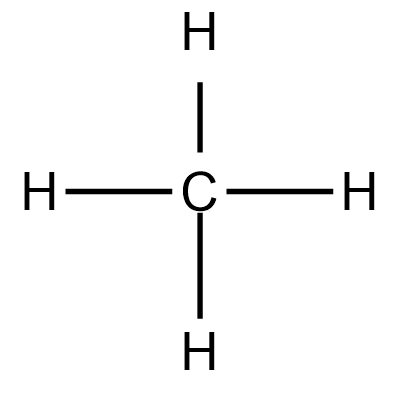# Problem: Consider three molecules: A, B, and C. Molecule A has a hybridization of sp 3. Molecule B has two more effective pairs (electron pairs around the central atom) than molecule A. Molecule C consists of two σ bonds and two π bonds. Give the molecular structure, hybridization, bond angles, and an example for each molecule.

###### FREE Expert Solution
• For molecule A, Recall that an sp3 hybridized atom has 4 regions of electron density. This electron density could either be a lone pair or bonding electrons.
• Methane (CH4) is a good example of this.
• C will appear as central atom with 4 Hs on it. Valence electrons of C is 4, H is 1. Total v.e is 16. 12+(4)1 = 16e-• CH4 follows a tetrahedral setup with 109.5bond angle.
83% (372 ratings)###### Problem Details

Consider three molecules: A, B, and C. Molecule A has a hybridization of sp 3. Molecule B has two more effective pairs (electron pairs around the central atom) than molecule A. Molecule C consists of two σ bonds and two π bonds. Give the molecular structure, hybridization, bond angles, and an example for each molecule.